# Complexity Explorer Santa Fe Institute

Your progress is not being saved! Enroll now or log in to track your progress or submit homework.## Introduction to Information Theory

• Measuring Information: Bits
• Adding Up Bits
• Using Bits to Count and Label
• Physical Forms of Information
• Fundamental Formula of Information
• Computation and Logic: Information Processing
• Mutual Information
• Communication Capacity in a Noisy Channel
• Shannon's Coding Theorem
• Homework Solutions

#### 4.1 Fundamental Formula of Information » Quiz Solutions

Question 1:

The formula for the number of sequences of m coin flips in which there are mh heads and mt tails is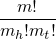.  Plugging in the values m=10 (there are 10 flips) and mh=7, mt=3 gives the number of sequences as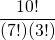.

By using a digital calculator (or simplifying in other ways), we can compute that=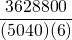=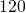.

Question 2:

From the video, remember the 'Fundamental formula of Information Theory’: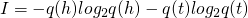, where q(h) is the probability of heads and q(t) is the probability of tails.

In this case, the probability of heads is q(h) = 0.6, so the probability of tails must be q(t) = 1-q(h) = 0.4.  Substituting these probabilities into the above equation gives
I = -0.6 log2 0.6 - 0.4 log2 0.4 ≈ 0.97 bits

Question 3:

Again we can use the remember the 'Fundamental formula of Information Theory’, though now for more than two possibilities: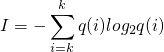,
where q(i) is the probability of possibility i.

If we have a fair six-sided die, then the probability of landing on each face is 1/6.  Plugging this into the above equation gives
I = - 6 * (1/6) log2 (1/6) = - log2 (1/6) = log2 6 ≈ 2.58 bits
(Here we have used the logarithmic identity -log 1/x = log x).

More generally, this is a demonstration of how choosing one of N options with uniform probability generates log2 N bits of information.

Question 4:

We again use the  'Fundamental formula of Information Theory’,,
where q(i) is the probability of possibility i.

We know that q(1) = 0.4.  That means that there remains 0.6 probability between the other 5 sides, so each of the remaining sides must have probability q(i) = 0.6/5 = 0.12 for i = 2...6.

Plugging these into the above formula gives
I = -0.4 log2 0.4 - 5*0.12 log2 0.12 ≈ 2.36 bits